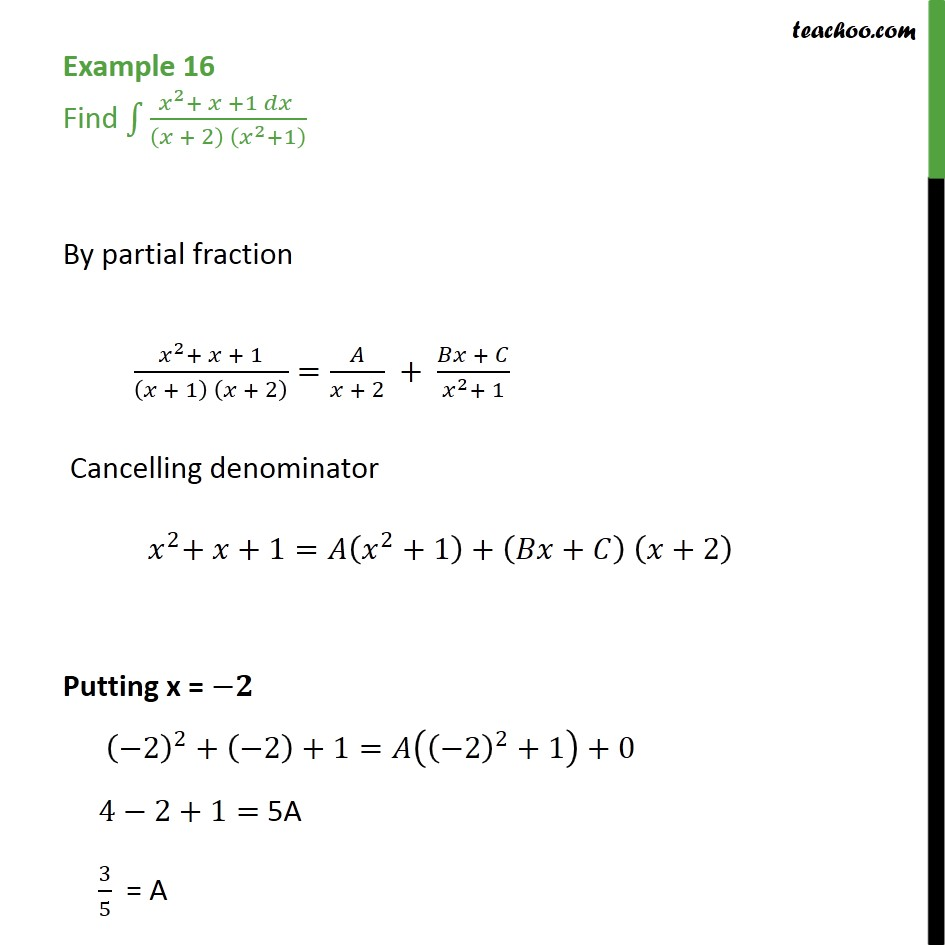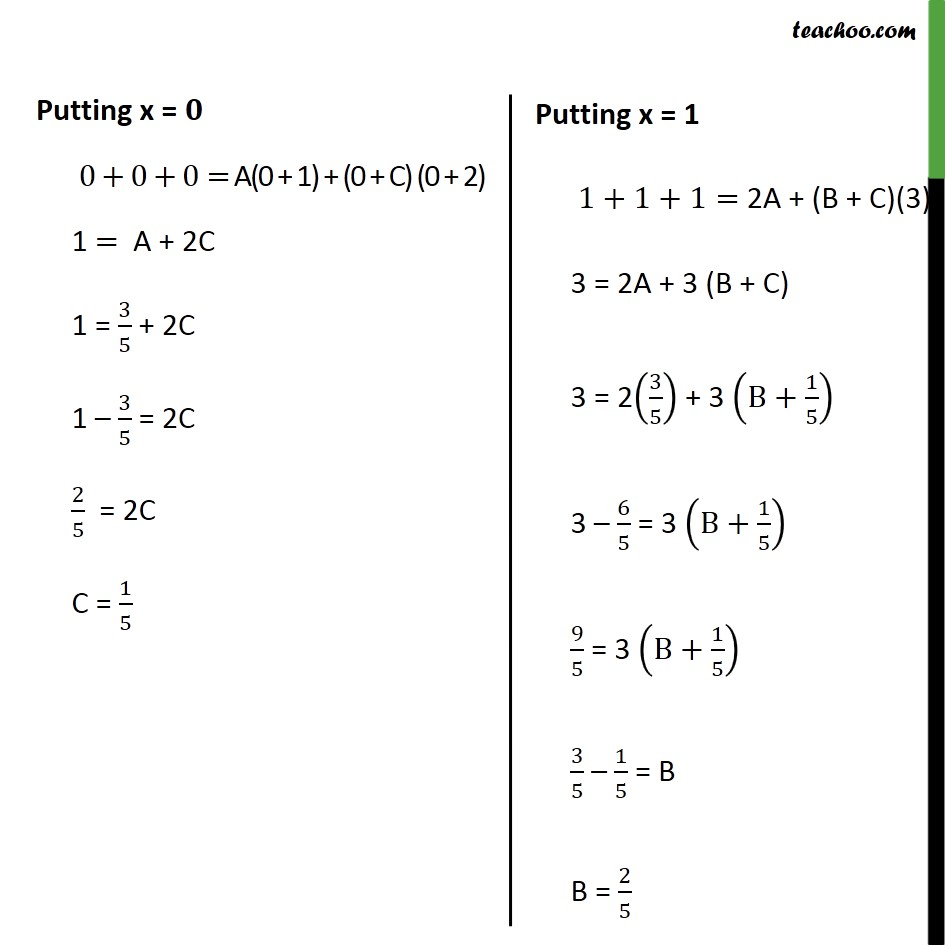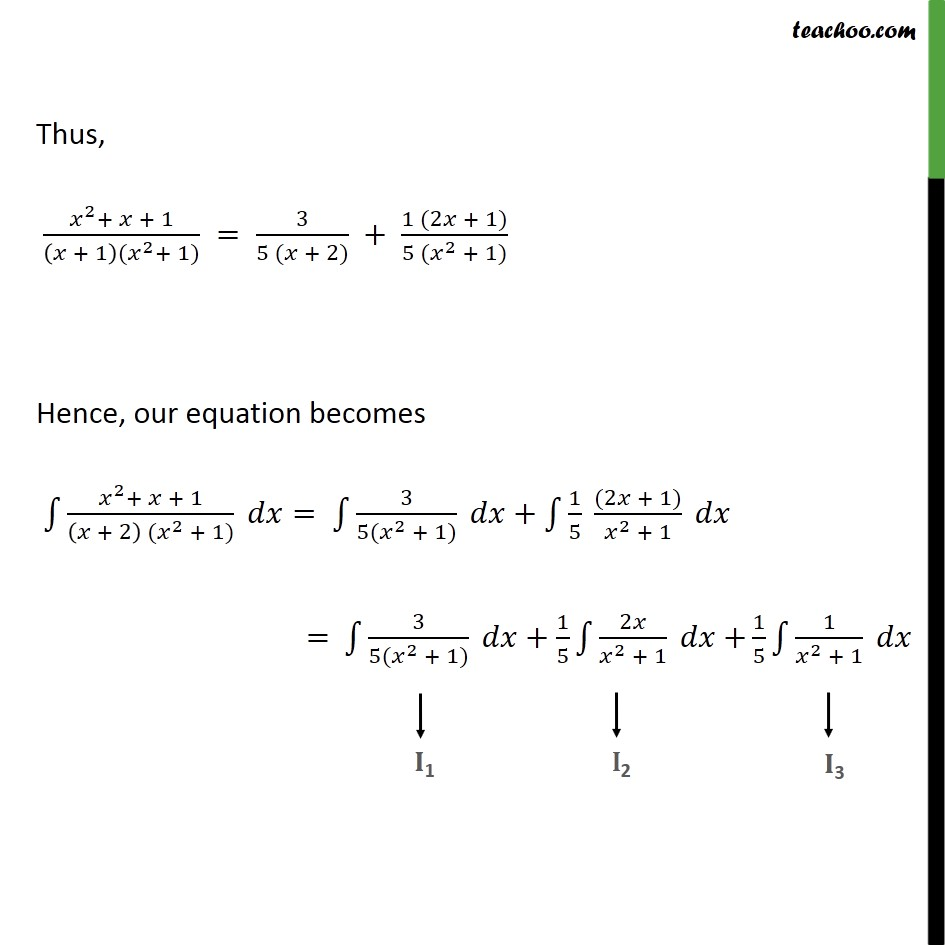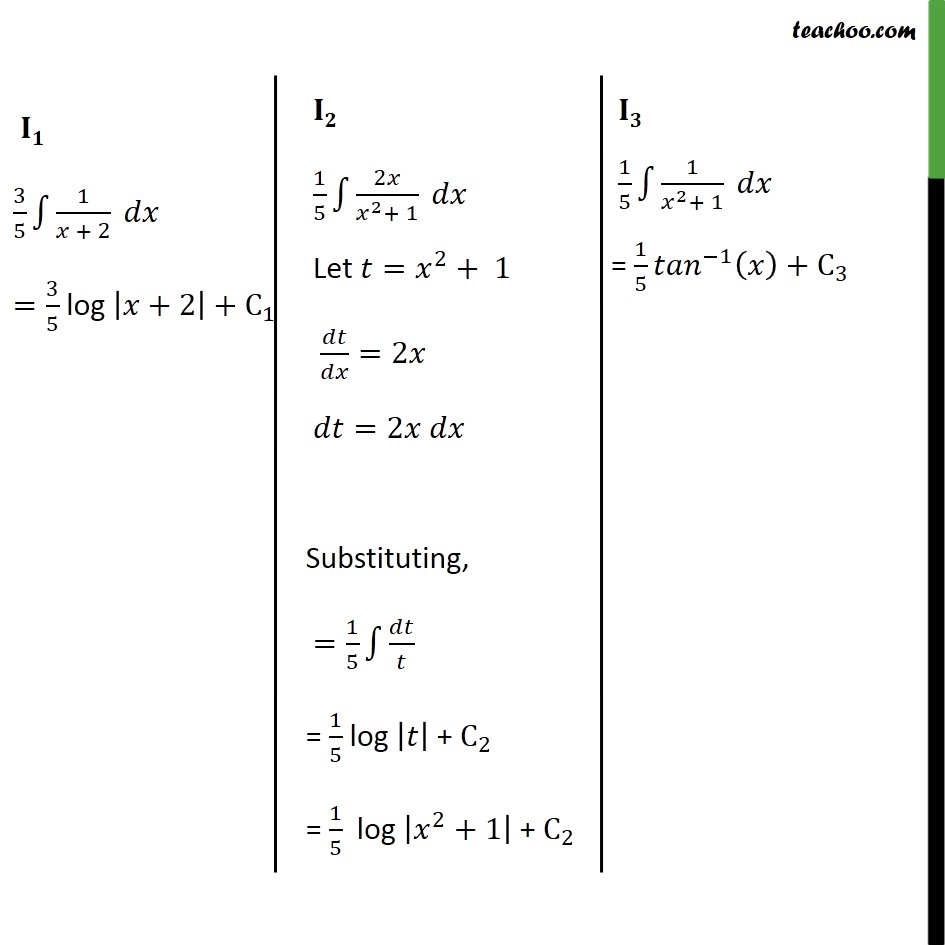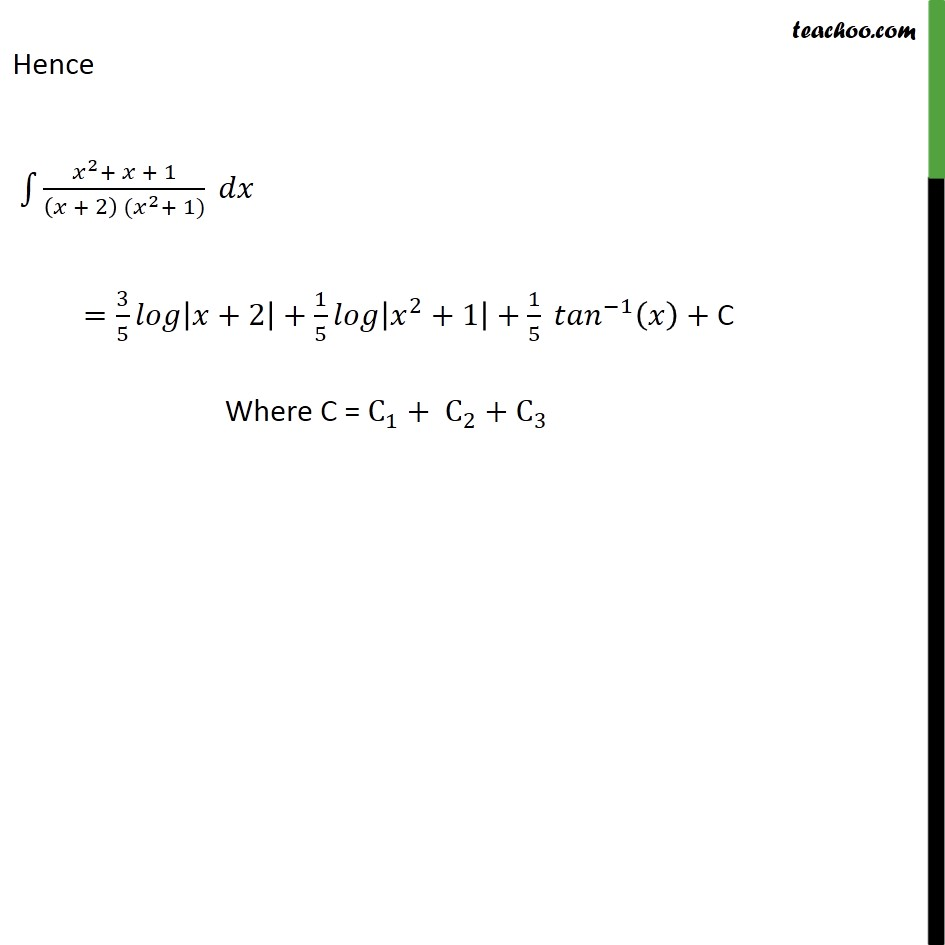1. Chapter 7 Class 12 Integrals
2. Concept wise
3. Integration by partial fraction - Type 5

Transcript

Example 16 Find 2 + +1 + 2 2 +1 By partial fraction 2 + + 1 + 1 + 2 = + 2 + + 2 + 1 Cancelling denominator 2 + +1= 2 +1 + + +2 Putting x = 2 2 + 2 +1= 2 2 +1 +0 4 2+1= 5A 3 5 = A Putting x = 0+0+0= A(0 + 1) + (0 + C) (0 + 2) 1 = A + 2C 1 = 3 5 + 2C 1 3 5 = 2C 2 5 = 2C C = 1 5 Thus, 2 + + 1 + 1 ( 2 + 1) = 3 5 ( + 2) + 1 (2 + 1) 5 ( 2 + 1) Hence, our equation becomes 2 + + 1 + 2 ( 2 + 1) = 3 5( 2 + 1) + 1 5 (2 + 1) 2 + 1 = 3 5( 2 + 1) + 1 5 2 2 + 1 + 1 5 1 2 + 1 Hence 2 + + 1 + 2 ( 2 + 1) = 3 5 +2 + 1 5 2 +1 + 1 5 1 + C Where C = C 1 + C 2 + C 3

Integration by partial fraction - Type 5